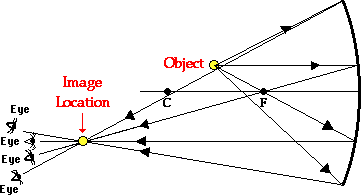Home » Posts tagged 'image'

# Tag Archives: image

## Now users at Ask Physics can add equations to their comments and questions.

Ask Physics has installed the Latex server and now users can type latex code to insert complex equations to their comments or questions.

If you are not familiar with latex code, use the following site to generate the code for the equation that you need.

http://www.codecogs.com/latex/eqneditor.php

When you visit the above site you can edit and view the result of the code generate. You have the option to copy the image of the equation or to copy the code itself (what you typed for getting the image equation; not the html code supplied) and paste the code in your comment.

Go, Try it Now!$(a+b)^{2}=a^{2}+b^{2}+2ab$

## How do we see an erect image though an inverted image is formed on the retina?

Our eye lens is a convex lens so an inverted image must be formed on our retina then how we see an erect image?

(Rahul Tyagi asks)

Answer:

What we see is the perception of the brain. Eye is for sensing and the seeing takes place at the Brain.

## A Question on EvaporationArunabh Arun sent this problem.

(Click on the image to see the enlarged version.)

## Optics MCQ – image formation by concave mirror

John Abraham asks:

I am having trouble with a problem.

Q. In an experiment to determine the focal length (f) of a concave mirror by the u–v method, a student places the object pin A on the principal axis at a distance x from the pole P. The student looks at the pin and its inverted image from a
distance keeping his/her eye in line with PA. When the student shifts his/her eye towards left, the image appears to the right of the object pin. Then

(A) x < f

(B) f < x < 2f

(C) x = 2f

(D) x > 2f

Answer:

The answer is B

(Try giving your explanation as comment. If not satisfied, ask again)EXPLANATION

The image and the object will move together at 2f

The one closer to the eye will move faster

In the question, the situation occurs when the image is closer to the eye and this happens when the object is between f and 2f

Hence the answer is B

## Five Sure Shot portions for SA2 (Class X CBSE)

The students appearing for SA2 of CBSE class X are requested to practice the following portions of Physics very well so that they can get excellently good marks in Science.

1. Practice all ray diagrams showing image formation by concave mirror, convex mirror, convex lens and concave lens along with characteristics of image.
2. Defects of eye, cause and rectification
3. Structure of human eye (diagram), function of each part, power of accommodation and persistence of vision.
4. Refraction through a glass slab and a glass prism, lateral displacement and angle of deviation.
5. Scattering of light, why sky is blue, Tyndal effect.
6. Also Practice numerical based on mirror formula, lens formula, refractive index.

## A numerical Problem from optics

AK asks:

When an object is kept at a distance of 60cm from a concave mirror, the magnification is 1/2. Where should the object be placed to get magnification of 1/3??

please answer this question….

Ans:

In the first part, u=-60, m=(-)1/2 implies v=-30 cm (Since the image must be real as concave lens form virtual image of bigger size only)

we get f = –20 cm

In II case

m=-1/3

v=u/3

f=-20cm

we get u=4f=4 x (-20)=-80 cm

(If this is not the expected answer please respond)

## Uses of diodes

Sajin John asks:

“What is the job of diode in a circuit? How can we recognize it?”

Answer:

A diode is a two terminal electronic device which allows passage of current only in one direction.A diode is used for rectification (converting ac to dc) and many other circuites

See the image of a diode on the left.

Read more on diodes at http://en.wikipedia.org/wiki/Diode

http://www.allaboutcircuits.com/vol_3/chpt_3/1.html

http://www.kpsec.freeuk.com/components/diode.htm

### Hits so far @ AskPhysics

• 2,271,288 hits

### Subscribe to AskPhysics via Email

Enter your email address to subscribe to this blog and receive notifications of new posts by email.

Join 4,336 other subscribers